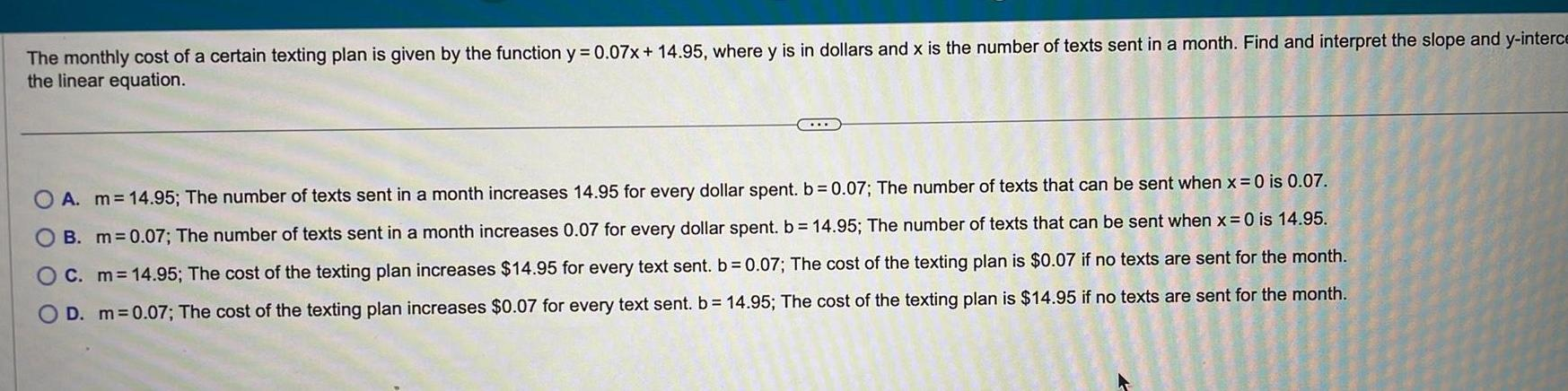Question:

# The monthly cost of a certain texting plan is given by the

Last updated: 9/18/2023The monthly cost of a certain texting plan is given by the function y 0 07x 14 95 where y is in dollars and x is the number of texts sent in a month Find and interpret the slope and y interce the linear equation A m 14 95 The number of texts sent in a month increases 14 95 for every dollar spent b 0 07 The number of texts that can be sent when x 0 is 0 07 B m 0 07 The number of texts sent in a month increases 0 07 for every dollar spent b 14 95 The number of texts that can be sent when x 0 is 14 95 OC m 14 95 The cost of the texting plan increases 14 95 for every text sent b 0 07 The cost of the texting plan is 0 07 if no texts are sent for the month O D m 0 07 The cost of the texting plan increases 0 07 for every text sent b 14 95 The cost of the texting plan is 14 95 if no texts are sent for the month C